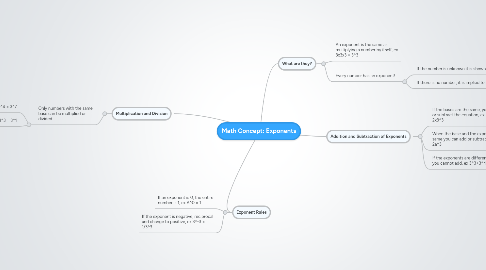# Math Concept: Exponents

Get Started. It's FreeMath Concept: Exponents## 2. Multiplication and Division

### 2.1. Only numbers with the same base can be multiplied or divided

2.1.1. Multiplication: 3^3 x 3^4 = 3^7

2.1.2. Division: 3^4 / 3^3 = 3^1

## 3. What are they?

### 3.2. Every number has an exponent!

3.2.1. If the number is unknown it is shown with n

3.2.2. If there is no number, it is implied to be 1